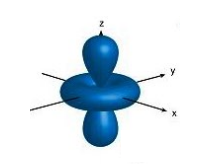# Problem: Which quantum numbers below could correctly describe the orbital to the right?        (a) n = 1, l = 0, m l = 0        (b) n = 2, l = 2, m l = 1        (c) n = 3, l = 1, m l = -1        (d) n = 3, l = 2, m l = -3        (e) n = 3, l = 2, m l = 2

###### FREE Expert Solution
80% (127 ratings)
###### Problem Details

Which quantum numbers below could correctly describe the orbital to the right?

(a) n = 1, l = 0, m l = 0

(b) n = 2, l = 2, m l = 1

(c) n = 3, l = 1, m l = -1

(d) n = 3, l = 2, m l = -3

(e) n = 3, l = 2, m l = 2